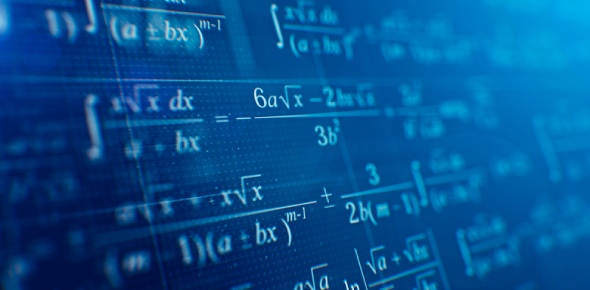# Exam 1 Math 3383

36 Questions | Total Attempts: 40Settings• 1.
A quadrilateral with opposite sides parallel
• 2.
A special parallelogram with four congruent sides
• 3.
A special parallelogram with four right angles
• 4.
An extra special parallelogram with four congruent sides and four right angles
• 5.
What two kinds of shapes are non-parallelograms?
• A.

Kite and Trapezoid

• B.

Square and Kite

• C.

Rhombus and Rectangle

• D.

Square and Trapezoid

• 6.
• 7.
A quadrilateral with one pair of parallel sides
• 8.
What three kinds of shapes are parallelograms?
• A.

Rhombus, Rectangle and Square

• B.

Trapezoid, Kite and Rhombus

• C.

Rectangle, Rhombus and Kite

• 9.
A closed 2D figure with no crossings or reuse of endpoints
• 10.
What is Euler's Formula?
• A.

V+E-2=F

• B.

F-2=V+E

• C.

V+F-2=E

• D.

E+F+V=2

• 11.
3D polyhedron with a flat base where triangular lateral faces meet at an apex
• 12.
3D polyhedron with two bases congruent to each other and parallel lateral faces
• 13.
Pyramid whose lateral edges are congruent
• 14.
Prism whose lateral edges meet at a right angle to the base
• 15.
What does it mean to be congruent?
• A.

Corresponding sides are congruent

• B.

Corresponding angles are congruent

• C.

No two sides have same length

• D.

At least two sides are same length

• 16.
Good conguence shortcuts
• A.

SSA

• B.

ASA

• C.

AAA

• D.

AAS

• E.

SSS

• F.

SAS

• 17.
A quadrilateral where non-parallel sides are conguent
• A.

Kite

• B.

Parallelogram

• C.

Rhombus

• D.

Isosceles Trapezoid

• 18.
What is the formula for the sum of interior angles of any polygon?
• A.

2-nx180

• B.

180-n+2

• C.

N-2x180

• D.

Nx180-2

• 19.
In any polygon, the number of sides=number of vertices=number of interior angles. In other words, the amount of each are the same.
• A.

True

• B.

False

• 20.
Find the sum of the interior angles in a dodecagon.
• 21.
Find the measure of each interior angle in an equiangular pentagon.
• 22.
What shapes are NON-POLYHEDRA?
• A.

Cylinder

• B.

Pyramid

• C.

Right pentagonal Prism

• D.

Cone

• 23.
In order to have a Regular Polygon, the shape has to be both...
• 24.
Line segment where two faces meet
• A.

Face

• B.

Base

• C.

Edge

• 25.
All squares are rectangles.
• A.

True

• B.

False

Related TopicsBack to top